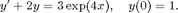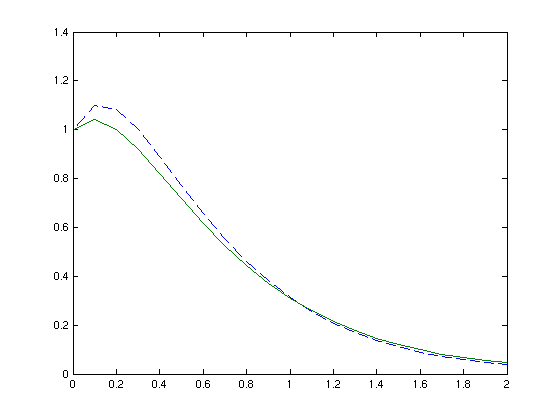# A simple example of publishing reports

## The Euler approximation of the differential equation on [0,2]:## Define the grid size, interval's partition, and the initial value

h=0.1;                       % grid size
x=0:h:2;                     % specify the partition of the interval [0,2]
clear y;                     % clear possibly existing variable y
y(1)=1;                      % initial condition: x1=0, thus y(1) corresponds to y(x1)=1


## Define the function

f=inline('3*exp(-4*x)-2*y');


## Compute the Euler approximation

size(x);                     % size of x to be used in determining the size of vector i
for i=1:20 y(i+1)=y(i)+h*f(x(i),y(i));end


## The exact solution

Y_exact=2.5*exp(-2*x)-1.5*exp(-4*x);


## The differences between exact and approximate solutions

error = abs(Y_exact-y)'

error =

0
0.0587
0.0793
0.0794
0.0696
0.0560
0.0418
0.0289
0.0180
0.0092
0.0025
0.0024
0.0058
0.0080
0.0093
0.0099
0.0100
0.0097
0.0092
0.0086
0.0079



## Plot of the exact and approximate (dashed curve) solutions

plot(x,y,'--',x,Y_exact)